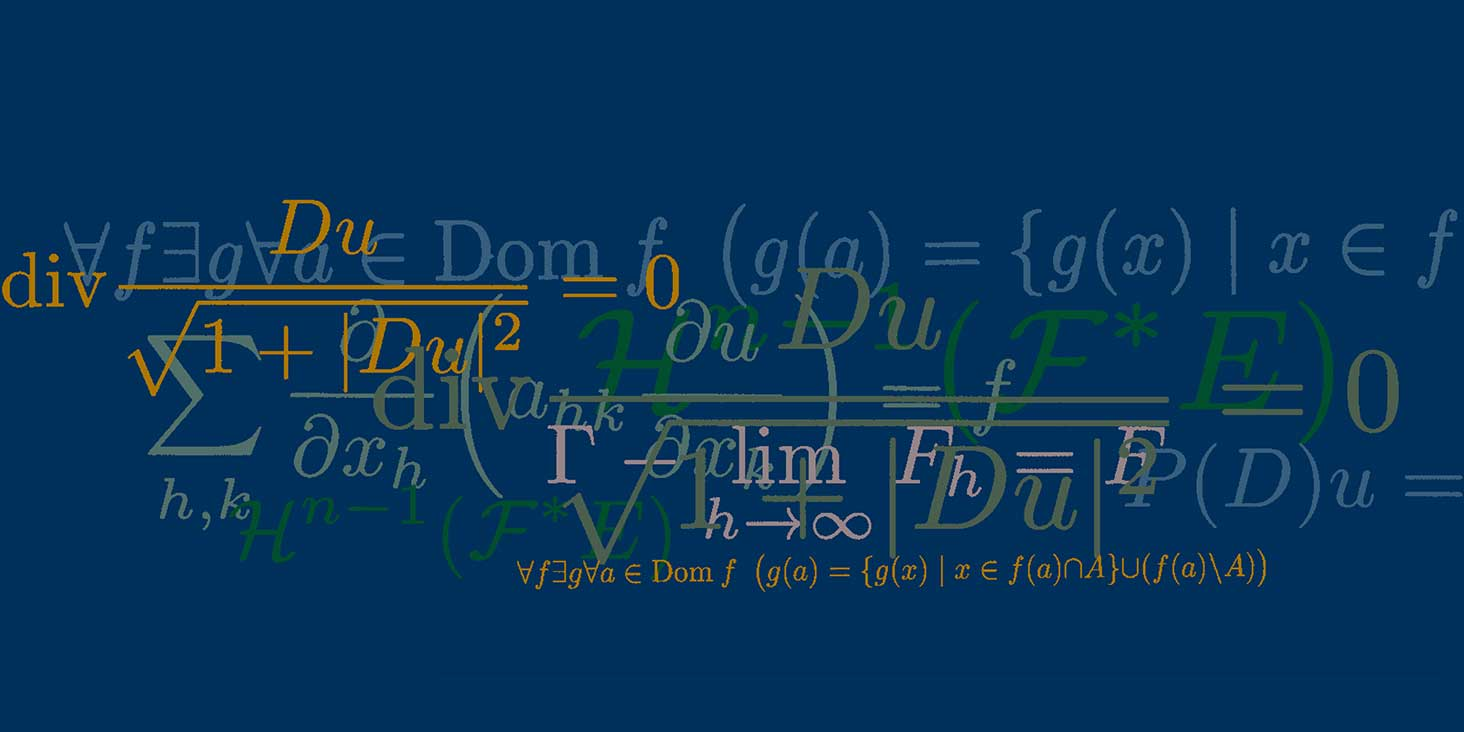# A Finiteness Theorem for Gromov-hyperbolic groups

Colloquio De Giorgi

## Speakers

Gérard Besson
Institut Fourier, CNRS and Université Grenoble Alpes

## Contatti

Gérard Besson - Institut Fourier, CNRS and Université Grenoble Alpes
A Finiteness Theorem for Gromov-hyperbolic  groups

Abstract
We shall prove that given $H>0$ and $\delta\geq 0$ there is a finite number (up to isomorphisms) of marked groups $(\Gamma , \Sigma)$ which are$\delta$-hyperbolic, torsion-free, non cyclic such that their entropy satisfies ${\rm Entropy} (\Gamma , \Sigma) \le H$. Here $\Gamma$ is finitely generated and endowed with a (symmetric) finite generating set $\Sigma$. The goal of the talk is to explain all the words in this abstract and give a flavour of the proof.

The event will take place in person.
Please note that, for organizational purposes, registration is mandatory.